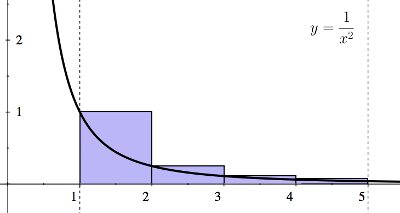# 009B Sample Midterm 2, Problem 1

Consider the region $S$bounded by $x=1,x=5,y={\frac {1}{x^{2}}}$and the $x$-axis.

a) Use four rectangles and a Riemann sum to approximate the area of the region $S$. Sketch the region $S$and the rectangles and
indicate whether your rectangles overestimate or underestimate the area of $S$.
b) Find an expression for the area of the region $S$as a limit. Do not evaluate the limit.Approximation of integral with left endpoints is an overestimate.
Foundations:
Recall:
1. The height of each rectangle in the left-hand Riemann sum is given by
choosing the left endpoint of the interval.
2. The height of each rectangle in the right-hand Riemann sum is given by
choosing the right endpoint of the interval.

Solution:

(a)

Step 1:
Let $f(x)={\frac {1}{x^{2}}}.$Since our interval is $[1,5]$and we are using $4$rectangles, each rectangle has width $1.$Since the problem doesn't specify, we can choose either right- or left-endpoints. Choosing left-endpoints, the Riemann sum is
$1\cdot (f(1)+f(2)+f(3)+f(4)).$Step 2:
Thus, the left-endpoint Riemann sum is
${\begin{array}{rcl}\displaystyle {1\cdot (f(1)+f(2)+f(3)+f(4))}&=&\displaystyle {{\bigg (}1+{\frac {1}{4}}+{\frac {1}{9}}+{1}{16}{\bigg )}}\\&&\\&=&\displaystyle {{\frac {205}{144}}.}\\\end{array}}$The left-endpoint Riemann sum overestimates the area of $S.$(b)

Step 1:
Let $n$be the number of rectangles used in the left-endpoint Riemann sum for $f(x)={\frac {1}{x^{2}}}.$The width of each rectangle is
$\Delta x={\frac {5-1}{n}}={\frac {4}{n}}.$Step 2:
So, the left-endpoint Riemann sum is
$\Delta x{\bigg (}f(1)+f{\bigg (}1+{\frac {4}{n}}{\bigg )}+f{\bigg (}1+2{\frac {4}{n}}{\bigg )}+\ldots +f{\bigg (}1+(n-1){\frac {4}{n}}{\bigg )}{\bigg )}.$Now, we let $n$go to infinity to get a limit.
So, the area of $S$is equal to
$\lim _{n\to \infty }{\frac {4}{n}}\sum _{i=0}^{n-1}f{\bigg (}1+i{\frac {4}{n}}{\bigg )}\,=\,\lim _{n\to \infty }{\frac {4}{n}}\sum _{i=0}^{n-1}{\bigg (}1+i{\frac {4}{n}}{\bigg )}^{-2}.$(a) The left-endpoint Riemann sum is ${\frac {205}{144}}$, which overestimates the area of $S$.
$\lim _{n\to \infty }{\frac {4}{n}}\sum _{i=0}^{n-1}{\bigg (}1+i{\frac {4}{n}}{\bigg )}^{-2}$